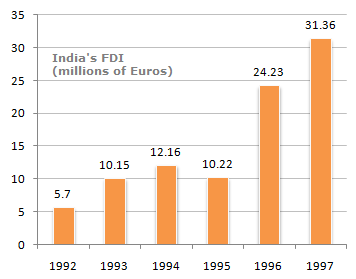# Data Interpretation - Bar Charts

Directions to Solve

The following bar chart shows the trends of foreign direct investments(FDI) into India from all over the world.

Trends of FDI in India1.
What was the ratio of investment in 1997 over the investment in 1992 ?
5.50
5.36
5.64
5.75
Explanation:
The 1997 figure of investment as a factor of 1992 investment = (31.36/5.70) = 5.50

2.
What was absolute difference in the FDI to India in between 1996 and 1997 ?
7.29
7.13
8.13
None of these
Explanation:

The difference in investments over 1996-1997 was

31.36 - 24.23 = € 7.13 millions.

3.
If India FDI from OPEC countries was proportionately the same in 1992 and 1997 as the total FDI from all over the world and if the FDI in 1992 from the OPEC countries was Euro 2 million. What was the amount of FDI from the OPEC countries in 1997 ?
11
10.72
11.28
11.5
Explanation:

Let x be the FDI in 1997.

Then: (2/5.7) = (x/31.36)

x = (2/5.7) x 31.36

x = 11

4.
Which year exhibited the highest growth in FDI in India over the period shown ?
1993
1994
1995
1996